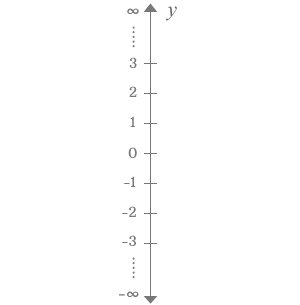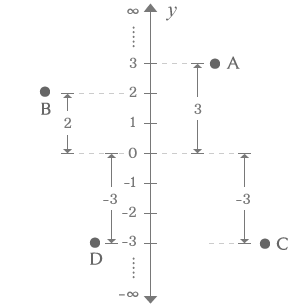y-axisA vertical number line that represents the vertical distances on a plane is called $y$-axis.

The y-axis is a number line and it is appeared perpendicular to the surface of the earth. Hence, it is also called as vertical axis. It contains numerous equal divisions and they are denoted by both positive and negative real numbers. The divisions which denoted by the numbers represent the vertical distances. It is mainly used to measure the vertical distance of different points on a plane in Cartesian coordinate system.

Parts

The vertical y-axis has three parts.

1.

Origin

The exact middle point of the y-axis is called origin and it is usually denoted by zero.

2.

Positive Region

The region above the origin is positive region of y-axis and it is divided into numerous divisions equally and each division is denoted by a positive number.

3.

Negative Region

The region below the origin is negative region of y-axis. It is also divided into numerous divisions equally but each division is indicated by a negative real number.

How to useA, B, C and D are four points. A and C points are appeared right side to y-axis but B and D points are appeared left side to y-axis. The points A and B are in positive region and C and D are in negative region.

The number line on the y-axis is used to compare the vertical distances of all four points. The vertical distances of all four points are measured from the origin of the y-axis.

1. The point A is at third division of y-axis from origin in positive region. Therefore, the vertical distance of point A is 3.
2. The point B is at second division of y-axis from origin in positive region. So, the vertical distance of point B is 2.
3. The point C is at third division of y-axis from origin but it is in negative region. So, the vertical distance of point C is -3.
4. The point D is also at third division of y-axis from origin and it is also in negative region. Therefore, the vertical distance of point D is also -3.

Latest Math Topics
Latest Math Problems
Email subscription
Math Doubts is a best place to learn mathematics and from basics to advanced scientific level for students, teachers and researchers. Know more